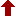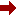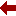#### SLIM21

 Sort Order Chronological Author Title ItemType,Author ItemType,Title Display Format Tabular Publication Bibliography Items / Page 5 10 25

Click the serial number on the left to view the details of the item.
#  AuthorTitleAccn#YearItem Type Claims
11 Schuch, Dieter Quantum Theory from a Nonlinear Perspective I10013 2018 eBook
12 Maximon, Leonard C Differential and Difference Equations I09655 2016 eBook
13 Papachristou, Costas J Aspects of Integrability of Differential Systems and Fields I09485 2019 eBook
14 Akhmet, Marat Bifurcation in Autonomous and Nonautonomous Differential Equations with Discontinuities I08930 2017 eBook
15 Tahir-Kheli, Raza Ordinary Differential Equations I08768 2018 eBook
16 Coley, A.A Dynamical Systems and Cosmology I11465 2003 eBook
17 Contopoulos, George Order and Chaos in Dynamical Astronomy I11280 2002 eBook
18 Vakakis, Alexander F Normal Modes and Localization in Nonlinear Systems I10997 2001 eBook
19 Lynch, Stephen Dynamical Systems with Applications using MAPLE I10751 2001 eBook
20 Kosmann-Schwarzbach, Yvette Integrability of Nonlinear Systems I10537 2004 eBook
 (page:2 / 11) [#101] First Page Previous Page Next Page Last Page

11.TitleQuantum Theory from a Nonlinear Perspective : Riccati Equations in Fundamental Physics
Author(s)Schuch, Dieter
PublicationCham, Springer International Publishing, 2018.
DescriptionXIV, 258 p. 15 illus., 7 illus. in color : online resource
Abstract NoteThis book provides a unique survey displaying the power of Riccati equations to describe reversible and irreversible processes in physics and, in particular, quantum physics. Quantum mechanics is supposedly linear, invariant under time-reversal, conserving energy and, in contrast to classical theories, essentially based on the use of complex quantities. However, on a macroscopic level, processes apparently obey nonlinear irreversible evolution equations and dissipate energy. The Riccati equation, a nonlinear equation that can be linearized, has the potential to link these two worlds when applied to complex quantities. The nonlinearity can provide information about the phase-amplitude correlations of the complex quantities that cannot be obtained from the linearized form. As revealed in this wide ranging treatment, Riccati equations can also be found in many diverse fields of physics from Bose-Einstein-condensates to cosmology. The book will appeal to graduate students and theoretical physicists interested in a consistent mathematical description of physical laws
ISBN,Price9783319655949
Keyword(s)1. Applications of Nonlinear Dynamics and Chaos Theory 2. DIFFERENTIAL EQUATIONS 3. EBOOK 4. EBOOK - SPRINGER 5. History and Philosophical Foundations of Physics 6. ORDINARY DIFFERENTIAL EQUATIONS 7. PHYSICS 8. QUANTUM PHYSICS 9. STATISTICAL PHYSICS
Item TypeeBook
Circulation Data
 Accession# Call# Status Issued To Return Due On Physical Location I10013 On Shelf

12.TitleDifferential and Difference Equations : A Comparison of Methods of Solution
Author(s)Maximon, Leonard C
PublicationCham, Springer International Publishing, 2016.
DescriptionXV, 162 p : online resource
Abstract NoteThis book, intended for researchers and graduate students in physics, applied mathematics and engineering, presents a detailed comparison of the important methods of solution for linear differential and difference equations - variation of constants, reduction of order, Laplace transforms and generating functions - bringing out the similarities as well as the significant differences in the respective analyses. Equations of arbitrary order are studied, followed by a detailed analysis for equations of first and second order. Equations with polynomial coefficients are considered and explicit solutions for equations with linear coefficients are given, showing significant differences in the functional form of solutions of differential equations from those of difference equations. An alternative method of solution involving transformation of both the dependent and independent variables is given for both differential and difference equations. A comprehensive, detailed treatment of Green???s functions and the associated initial and boundary conditions is presented for differential and difference equations of both arbitrary and second order. A dictionary of difference equations with polynomial coefficients provides a unique compilation of second order difference equations obeyed by the special functions of mathematical physics. Appendices augmenting the text include, in particular, a proof of Cramer???s rule, a detailed consideration of the role of the superposition principal in the Green???s function, and a derivation of the inverse of Laplace transforms and generating functions of particular use in the solution of second order linear differential and difference equations with linear coefficients
ISBN,Price9783319297361
Keyword(s)1. APPLIED MATHEMATICS 2. Difference and Functional Equations 3. DIFFERENCE EQUATIONS 4. DIFFERENTIAL EQUATIONS 5. EBOOK 6. EBOOK - SPRINGER 7. ENGINEERING MATHEMATICS 8. FUNCTIONAL EQUATIONS 9. Mathematical and Computational Engineering 10. Mathematical Applications in the Physical Sciences 11. Mathematical Methods in Physics 12. MATHEMATICAL PHYSICS 13. ORDINARY DIFFERENTIAL EQUATIONS 14. PHYSICS
Item TypeeBook
Circulation Data
 Accession# Call# Status Issued To Return Due On Physical Location I09655 On Shelf

13.TitleAspects of Integrability of Differential Systems and Fields : A Mathematical Primer for Physicists
Author(s)Papachristou, Costas J
PublicationCham, Springer International Publishing, 2019.
DescriptionVIII, 94 p. 20 illus : online resource
Abstract NoteThis book serves as an introduction to the concept of integrability as it applies to systems of differential equations as well as to vector-valued fields. The author focuses on specific aspects of integrability that are often encountered in a variety of problems in applied mathematics, physics and engineering. The following general cases of integrability are examined: (a) path-independence of line integrals of vector fields on the plane and in space; (b) integration of a system of ordinary differential equations by using first integrals; and (c) integrable systems of partial differential equations. Special topics include the integration of analytic functions and some elements from the geometric theory of differential systems. Certain more advanced subjects, such as Lax pairs and B??cklund transformations, are also discussed. The book is written at an intermediate level for educational purposes. The presentation is as simple as the topics allow, often sacrificing mathematical rigor in favor of pedagogical efficiency
ISBN,Price9783030350024
Keyword(s)1. DIFFERENTIAL EQUATIONS 2. EBOOK 3. EBOOK - SPRINGER 4. Mathematical Methods in Physics 5. MATHEMATICAL PHYSICS 6. ORDINARY DIFFERENTIAL EQUATIONS 7. PARTIAL DIFFERENTIAL EQUATIONS 8. PHYSICS
Item TypeeBook
Circulation Data
 Accession# Call# Status Issued To Return Due On Physical Location I09485 On Shelf

14.TitleBifurcation in Autonomous and Nonautonomous Differential Equations with Discontinuities
Author(s)Akhmet, Marat;Kashkynbayev, Ardak
PublicationSingapore, Springer Singapore, 2017.
DescriptionXI, 166 p. 31 illus., 26 illus. in color : online resource
Abstract NoteThis book is devoted to bifurcation theory for autonomous and nonautonomous differential equations with discontinuities of different types. That is, those with jumps present either in the right-hand-side or in trajectories or in the arguments of solutions of equations. The results obtained in this book can be applied to various fields such as neural networks, brain dynamics, mechanical systems, weather phenomena, population dynamics, etc. Without any doubt, bifurcation theory should be further developed to different types of differential equations. In this sense, the present book will be a leading one in this field. The reader will benefit from the recent results of the theory and will learn in the very concrete way how to apply this theory to differential equations with various types of discontinuity. Moreover, the reader will learn new ways to analyze nonautonomous bifurcation scenarios in these equations. The book will be of a big interest both for ??beginners and experts in the field. For the former group of specialists, that is, undergraduate and graduate students, the book will be useful since it provides a strong impression that bifurcation theory can be developed not only for discrete and continuous systems, but those which combine these systems in very different ways. The latter group of specialists will find in this book several powerful instruments developed for the theory of discontinuous dynamical systems with variable moments of impacts, differential equations with piecewise constant arguments of generalized type and Filippov systems. A significant benefit of the present book is expected to be for those who consider bifurcations in systems with impulses since they are presumably nonautonomous systems
ISBN,Price9789811031809
Keyword(s)1. Applications of Nonlinear Dynamics and Chaos Theory 2. Control and Systems Theory 3. Control engineering 4. Difference and Functional Equations 5. DIFFERENCE EQUATIONS 6. DIFFERENTIAL EQUATIONS 7. Dynamical Systems and Ergodic Theory 8. DYNAMICS 9. EBOOK 10. EBOOK - SPRINGER 11. ERGODIC THEORY 12. FUNCTIONAL EQUATIONS 13. ORDINARY DIFFERENTIAL EQUATIONS 14. STATISTICAL PHYSICS
Item TypeeBook
Circulation Data
 Accession# Call# Status Issued To Return Due On Physical Location I08930 On Shelf

15.TitleOrdinary Differential Equations : Mathematical Tools for Physicists
Author(s)Tahir-Kheli, Raza
PublicationCham, Springer International Publishing, 2018.
DescriptionXXII, 408 p. 65 illus., 1 illus. in color : online resource
Abstract NoteThis textbook describes rules and procedures for the use of Differential Operators (DO) in Ordinary Differential Equations (ODE ). The book provides a detailed theoretical and numerical description of ODE. It presents a large variety of ODE and the chosen groups are used to solve a host of physical problems. Solving these problems is of interest primarily to students of science, such as physics, engineering, biology and chemistry. Scientists are greatly assisted by using the DO obeying several simple algebraic rules. The book describes these rules and, to help the reader, the vocabulary and the definitions used throughout the text are provided. A thorough description of the relatively straightforward methodology for solving ODE is given. The book provides solutions to a large number of associated problems. ODE that are integrable, or those that have one of the two variables missing in any explicit form are also treated with solved problems. The physics and applicable mathematics are explained and many associated problems are analyzed and solved in detail. Numerical solutions are analyzed and the level of exactness obtained under various approximations is discussed in detail.
ISBN,Price9783319764061
Keyword(s)1. DIFFERENTIAL EQUATIONS 2. EBOOK 3. EBOOK - SPRINGER 4. Mathematical Applications in the Physical Sciences 5. Mathematical Methods in Physics 6. MATHEMATICAL PHYSICS 7. MECHANICS 8. Mechanics, Applied 9. ORDINARY DIFFERENTIAL EQUATIONS 10. PHYSICS 11. Theoretical and Applied Mechanics
Item TypeeBook
Circulation Data
 Accession# Call# Status Issued To Return Due On Physical Location I08768 On Shelf

16.TitleDynamical Systems and Cosmology
Author(s)Coley, A.A
PublicationDordrecht, Springer Netherlands, 2003.
DescriptionVIII, 195 p : online resource
Abstract NoteDynamical systems theory is especially well-suited for determining the possible asymptotic states (at both early and late times) of cosmological models, particularly when the governing equations are a finite system of autonomous ordinary differential equations. In this book we discuss cosmological models as dynamical systems, with particular emphasis on applications in the early Universe. We point out the important role of self-similar models. We review the asymptotic properties of spatially homogeneous perfect fluid models in general relativity. We then discuss results concerning scalar field models with an exponential potential (both with and without barotropic matter). Finally, we discuss the dynamical properties of cosmological models derived from the string effective action. This book is a valuable source for all graduate students and professional astronomers who are interested in modern developments in cosmology
ISBN,Price9789401703277
Keyword(s)1. Applications of Mathematics 2. APPLIED MATHEMATICS 3. Astronomy, Observations and Techniques 4. Astronomy???Observations 5. Classical and Quantum Gravitation, Relativity Theory 6. COSMOLOGY 7. DIFFERENTIAL EQUATIONS 8. EBOOK 9. EBOOK - SPRINGER 10. ENGINEERING MATHEMATICS 11. GRAVITATION 12. MATHEMATICAL PHYSICS 13. Observations, Astronomical 14. ORDINARY DIFFERENTIAL EQUATIONS 15. Theoretical, Mathematical and Computational Physics
Item TypeeBook
Circulation Data
 Accession# Call# Status Issued To Return Due On Physical Location I11465 On Shelf

17.TitleOrder and Chaos in Dynamical Astronomy
Author(s)Contopoulos, George
PublicationBerlin, Heidelberg, Springer Berlin Heidelberg, 2002.
DescriptionXIII, 624 p : online resource
Abstract NoteThe study of orbits in dynamical systems and the theory of order and chaos has progressed enormously over the last few decades. It thus became an essential tool in dynamical astronomy. The book is the first to provide a general overview of order and chaos in dynamical astronomy. The progress of the theory of chaos has a profound impact on galactic dynamics. It has even invaded celestial mechanics, since chaos was found in the solar system which in the past was considered as a prototype of order. The book provides a unifying approach to these topics from an author who has spent more than 50 years of research in the field. The first part treats order and chaos in general. The other two parts deal with order and chaos in galaxies and with other applications in dynamical astronomy, ranging from celestial mechanics to general relativity and cosmology. This book, addressing especially the astrophysics, is also written as a textbook on dynamical systems for students in physics
ISBN,Price9783662049174
Keyword(s)1. ASTRONOMY 2. Astronomy, Astrophysics and Cosmology 3. ASTROPHYSICS 4. Astrophysics and Astroparticles 5. COMPLEX SYSTEMS 6. DIFFERENTIAL EQUATIONS 7. DYNAMICAL SYSTEMS 8. Dynamical Systems and Ergodic Theory 9. DYNAMICS 10. EBOOK 11. EBOOK - SPRINGER 12. ERGODIC THEORY 13. MATHEMATICAL PHYSICS 14. ORDINARY DIFFERENTIAL EQUATIONS 15. STATISTICAL PHYSICS 16. Theoretical, Mathematical and Computational Physics
Item TypeeBook
Circulation Data
 Accession# Call# Status Issued To Return Due On Physical Location I11280 On Shelf

18.TitleNormal Modes and Localization in Nonlinear Systems
Author(s)Vakakis, Alexander F
PublicationDordrecht, Springer Netherlands, 2001.
DescriptionVI, 294 p : online resource
Abstract NoteThe nonlinear normal modes of a parametrically excited cantilever beam are constructed by directly applying the method of multiple scales to the governing integral-partial differential equation and associated boundary conditions. The effect of the inertia and curvature nonlin?? earities and the parametric excitation on the spatial distribution of the deflection is examined. The results are compared with those obtained by using a single-mode discretization. In the absence of linear viscous and quadratic damping, it is shown that there are nonlinear normal modes, as defined by Rosenberg, even in the presence of a principal parametric excitation. Furthermore, the nonlinear mode shape obtained with the direct approach is compared with that obtained with the discretization approach for some values of the excitation frequency. In the single-mode discretization, the spatial distribution of the deflection is assumed a priori to be given by the linear mode shape ??n, which is parametrically excited, as Equation (41). Thus, the mode shape is not influenced by the nonlinear curvature and nonlinear damping. On the other hand, in the direct approach, the mode shape is not assumed a priori; the nonlinear effects modify the linear mode shape ??n. Therefore, in the case of large-amplitude oscillations, the single-mode discretization may yield inaccurate mode shapes. References 1. Vakakis, A. F., Manevitch, L. I., Mikhlin, Y. v., Pilipchuk, V. N., and Zevin A. A., Nonnal Modes and Localization in Nonlinear Systems, Wiley, New York, 1996
ISBN,Price9789401724524
Keyword(s)1. CLASSICAL MECHANICS 2. DIFFERENTIAL EQUATIONS 3. DYNAMICAL SYSTEMS 4. DYNAMICS 5. EBOOK 6. EBOOK - SPRINGER 7. MECHANICS 8. ORDINARY DIFFERENTIAL EQUATIONS 9. PARTIAL DIFFERENTIAL EQUATIONS 10. VIBRATION 11. Vibration, Dynamical Systems, Control
Item TypeeBook
Circulation Data
 Accession# Call# Status Issued To Return Due On Physical Location I10997 On Shelf

19.TitleDynamical Systems with Applications using MAPLE
Author(s)Lynch, Stephen
PublicationBoston, MA, 1. Birkh??user Boston 2. Imprint: Birkh??user, 2001.
DescriptionXIII, 399 p. 83 illus : online resource
Abstract Note"The text treats a remarkable spectrum of topics and has a little for everyone. It can serve as an introduction to many of the topics of dynamical systems, and will help even the most jaded reader, such as this reviewer, enjoy some of the interactive aspects of studying dynamics using Maple." ???UK Nonlinear News (Review of First Edition) "The book will be useful for all kinds of dynamical systems courses???. [It] shows the power of using a computer algebra program to study dynamical systems, and, by giving so many worked examples, provides ample opportunity for experiments. ??? [It] is well written and a pleasure to read, which is helped by its attention to historical background." ???Mathematical Reviews (Review of First Edition) Since the first edition of this book was published in 2001, Maple??? has evolved from Maple V into Maple 13. Accordingly, this new edition has been thoroughly updated and expanded to include more applications, examples, and exercises, all with solutions; two new chapters on neural networks and simulation have also been added. There are also new sections on perturbation methods, normal forms, Gr??bner bases, and chaos synchronization. The work provides an introduction to the theory of dynamical systems with the aid of Maple. The author has emphasized breadth of coverage rather than fine detail, and theorems with proof are kept to a minimum. Some of the topics treated are scarcely covered elsewhere. Common themes such as bifurcation, bistability, chaos, instability, multistability, and periodicity run through several chapters. The book has a hands-on approach, using Maple as a pedagogical tool throughout. Maple worksheet files are listed at the end of each chapter, and along with commands, programs, and output may be viewed in color at the author???s website. Additional applications and further links of interest may be found at Maplesoft???s Application Center. Dynamical Systems with Applications using Maple is aimed at senior undergraduates, graduate students, and working scientists in various branches of applied mathematics, the natural sciences, and engineering. ISBN 978-0-8176-4389-8 ?? Also by the author: Dynamical Systems with Applications using MATLAB??, ISBN 978-0-8176-4321-8 Dynamical Systems with Applications using Mathematica??, ISBN 978-0-8176-4482-6
ISBN,Price9781489928498
Keyword(s)1. Applications of Mathematics 2. APPLIED MATHEMATICS 3. COMPLEXITY 4. COMPUTATIONAL COMPLEXITY 5. Computational Mathematics and Numerical Analysis 6. Computer mathematics 7. DIFFERENTIAL EQUATIONS 8. EBOOK 9. EBOOK - SPRINGER 10. ENGINEERING MATHEMATICS 11. Mathematical Modeling and Industrial Mathematics 12. MATHEMATICAL MODELS 13. MATHEMATICAL PHYSICS 14. ORDINARY DIFFERENTIAL EQUATIONS 15. Theoretical, Mathematical and Computational Physics
Item TypeeBook
Circulation Data
 Accession# Call# Status Issued To Return Due On Physical Location I10751 On Shelf

20.TitleIntegrability of Nonlinear Systems
Author(s)Kosmann-Schwarzbach, Yvette;Grammaticos, Basil;Tamizhmani, K.M
PublicationBerlin, Heidelberg, Springer Berlin Heidelberg, 2004.
DescriptionXII, 340 p : online resource
Abstract NoteThe lectures that comprise this volume constitute a comprehensive survey of the many and various aspects of integrable dynamical systems. The present edition is a streamlined, revised and updated version of a 1997 set of notes that was published as Lecture Notes in Physics, Volume 495. This volume will be complemented by a companion book dedicated to discrete integrable systems. Both volumes address primarily graduate students and nonspecialist researchers but will also benefit lecturers looking for suitable material for advanced courses and researchers interested in specific topics
ISBN,Price9783540409625
Keyword(s)1. COMPLEX SYSTEMS 2. DIFFERENTIAL EQUATIONS 3. DYNAMICAL SYSTEMS 4. EBOOK 5. EBOOK - SPRINGER 6. Mathematical Methods in Physics 7. ORDINARY DIFFERENTIAL EQUATIONS 8. PARTIAL DIFFERENTIAL EQUATIONS 9. PHYSICS 10. STATISTICAL PHYSICS 11. Statistical Physics and Dynamical Systems
Item TypeeBook